# Chapter Notes: Introduction to Numbers Notes | Study Mathematics for Class 4 - Class 4

## Class 4: Chapter Notes: Introduction to Numbers Notes | Study Mathematics for Class 4 - Class 4

The document Chapter Notes: Introduction to Numbers Notes | Study Mathematics for Class 4 - Class 4 is a part of the Class 4 Course Mathematics for Class 4.
All you need of Class 4 at this link: Class 4

• At the time of Independence, India had 23,444 post offices all over the country. Today, we have more than 1,55,618 post offices.
• Can you read the above numbers?Were you able to read the above numbers? Don't worry we will tell you!

How to Read and Write 5-Digit Numbers?

• We know that 9,999 is the largest 4-digit number. If we add 1 to it, we get 10,000, which is the smallest 5-digit number.
• This also leads to a new place called the ten thousands place. 10,000 is read as ten thousand.
Look at the following books:• There are 10 books with 1,000 pages in each book.
• We say that there are 10 thousands = 1 ten thousand = 10,000 pages in all.
• Thus, 10 thousands = 1 ten thousand = 10,000.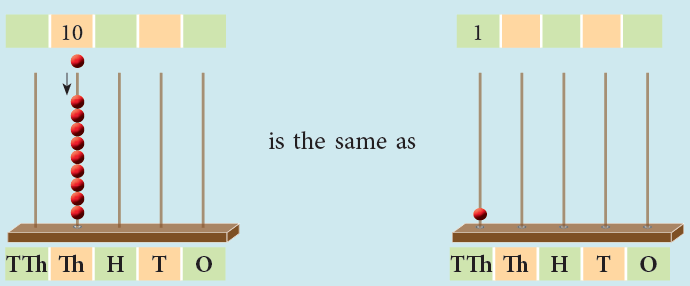Instructions to Follow

1. Count by Ten thousands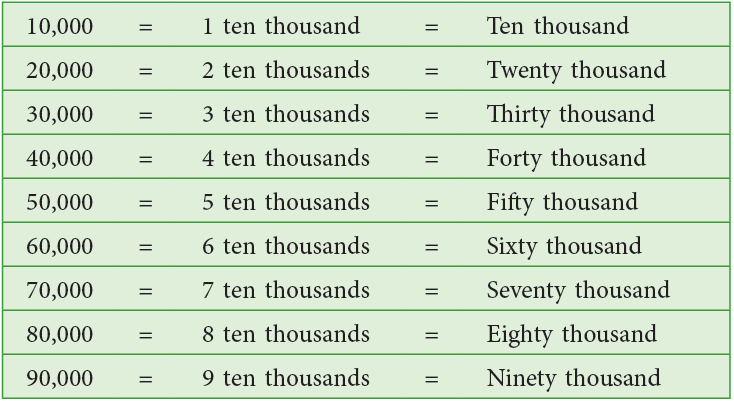2. Forming 5-Digit Numbers

• If we add 1 to the smallest 5-digit number, 10,000, we get
10,000 + 1 = 10,001 (which is read as ten thousand one).
• If we add 1 to 10,001, we get 10,001 + 1 = 10,002 (which is read as ten thousand two),...
• 99,998 + 1 = 99,999 (which is read as ninety-nine thousand nine hundred ninety-nine).
• 99,999 is the greatest 5-digit number.
• If we add 1 to this number, we get 99,999 + 1 = 1,00,000 which is read as 100 thousands or 1 lakh.
This number has 6 digits.
• 1,00,000 is the smallest 6-digit number.

Edurev Tips:

• Ones Period: Ones, Tens, Hundreds
• Thousands Period: Thousands, Ten Thousands

Try yourself:Which of the following is the smallest 5-digit number?

3. Writing 5-Digit Numbers

• We split up a large number into groups or periods starting from the right to make it easy to read and write.
• For a 5-digit number, the first three places from the right make the ones period and the next two make the thousands period. We separate the periods using commas or leave a short space.
• Thus, the number 18730 can be written as 18,730 or 18 730.

• While reading a number, all the digits in the same period are read together and the name of the period (except the ones) is read with the digits.
• Let us read the number 36,207.
• We arrange the digits of given number in the place value chart, as shown below.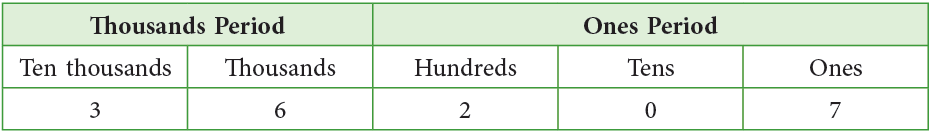We have, 36,207 = Thirty-six thousand two hundred seven.
Similarly, 50,327 = Fifty thousand three hundred twenty-seven.
• We can represent the above numbers on the abacus, as shown below: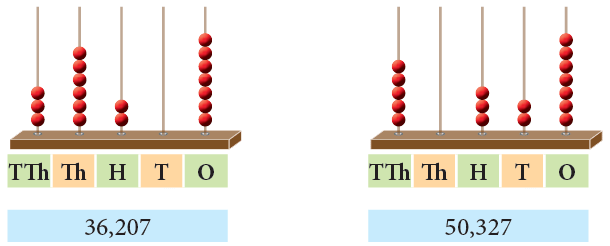How to Read and Write 6-Digit Numbers?

To write the smallest 6-digit number, 1 lakh or 100000 using commas, we proceed as under:

• Starting from the right, we put a comma after three digits and then another comma after the fifth digit.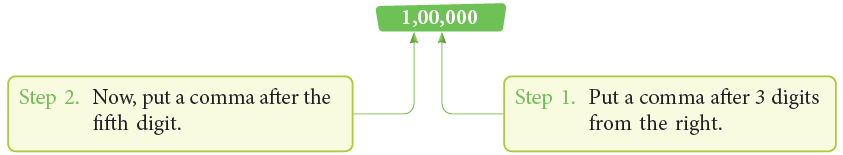We represent the smallest 6-digit number 1,00,000, in the Indian place value chart, as shown below: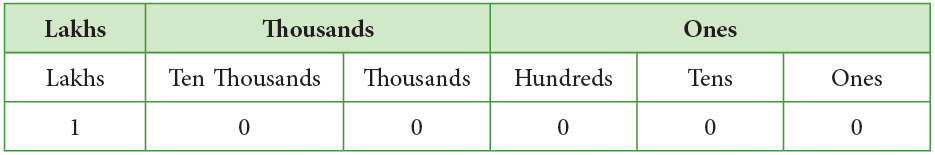1 is in the lakhs place. So, 1,00,000 is read as 1 lakh.

Instructions to Follow

1. Counting in Lakhs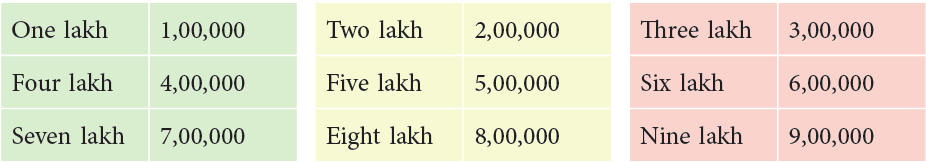2. Forming 6-Digit Numbers

• 6-digit numbers beyond 1,00,000 can be formed as follows.
1,00,000 + 1 = 1,00,001 (One lakh one);
1,00,001 + 1 = 1,00,002 (One lakh two);
1,11,110 + 1 = 1,11,111 (One lakh eleven thousand one hundred eleven),…,
9,99,998 + 1 = 9,99,999 (Nine lakh ninety-nine thousand nine hundred ninety-nine);
9,99,999 is the greatest 6-digit number.
• In a 6-digit number, the first three places from the right make the ones period, the next two make the thousands period and the next, that is, the sixth place, makes the lakhs period.
• We may put a comma between different periods or leave space between them. Thus, ‘four lakh’ may be written as 4,00,000 or 4 00 000.

3. Representing 6-Digit Numbers on Abacus

• We can represent 6-digit numbers on the abacus, as follows.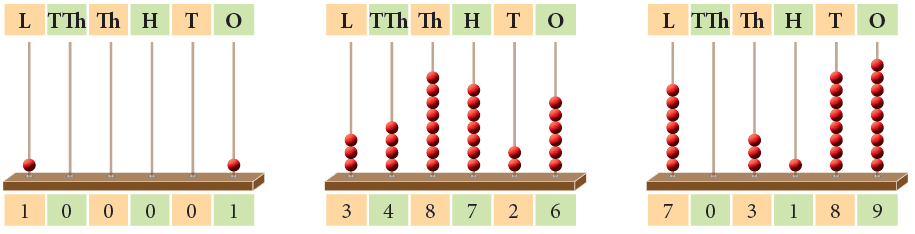• The abacuses shown above represent the numbers 1,00,001 and 3,48,726 and 7,03,189, respectively.
• They are read as one lakh one; three lakh forty-eight thousand seven hundred twenty-six and seven lakh three thousand one hundred eighty-nine, respectively.

What is Face Value and Place Value?

• The face value of a digit is the actual value of the digit itself, irrespective of its position in a number.
• The place value of a digit in a given number is its value due to its place or position in the number.
• Consider the numbers 81,246 and 1,34,279.
• Here, the place values of 4 are 4 tens (= 40) and 4 thousands (4,000), respectively.
The face value of 4 is 4 in both the numbers.

Edurev Tips:

• Place value = Face value × value of the place
• The place value and face value of 0 is always 0.

Try yourself:Which of the following is true for the number 82,951?

Example 1: Write the face value and place value of each digit in 1 72 827.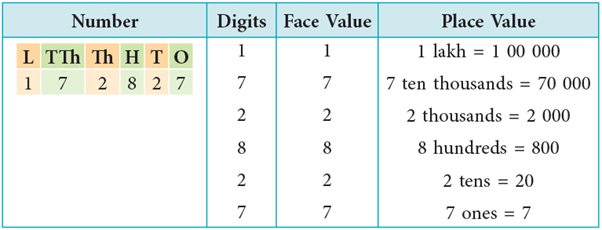How to Write in Expanded Notation?

The number 23,344 in the expanded notation is written as:
23,344 = 2 ten thousands + 3 thousands + 3 hundreds + 4 tens + 4 ones
= 20,000 + 3,000 + 300 + 40 + 4
Let us consider the number 4,78,362 in the expanded notation.
4,78,362 = 4 lakhs + 7 ten thousands + 8 thousands + 3 hundreds + 6 tens + 2 ones
= 4,00,000 + 70,000 + 8,000 + 300 + 60 + 2

Edurev Tips: In expanded notation, a number is written as the sum of the place values of its digits.

How to Compare and Arrange Numbers?

Case 1: Comparing Numbers with Different Number of Digits

Rule: A number containing greater number of digits is larger than a number containing lesser number of digits.

54,207 > 8,964
(5 digits)   (4 digits)

Example 1: Which is the greatest: 3,815 or 567 or 36,812?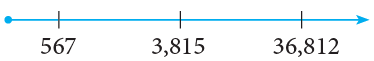If you write these numbers on the number line, the number 567 will come first, then 3,815 and then 36,812, from the left to right. Since 567 is to the left of 3,815, therefore, 567 < 3,815 or 3,815 > 567.
Also, since 3,815 is to the left of 36,812, therefore, 3,815 < 36,812 or 36,812 > 3,815.
Therefore, 36,812 is the greatest.

Case 2: Comparing Numbers with Same Numbers of Digits

Rule: To compare numbers with equal number of digits, start by comparing the digits from the leftmost place till you find two different digits. Compare these digits to decide the greater and smaller of the given numbers.

Example 1: Which is greater: 32,719 or 45,989?

Both are 5-digit numbers but the number 32,719 has 3 ten thousands, while the number 45,989 has 4 ten thousands.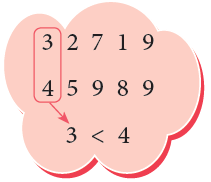Since 4 ten thousands is greater than 3 ten thousands, therefore, 45,989 > 32,719.

Example 2: Which is greater: 7,32,612 or 7,32,545?

Both the numbers are 6-digit numbers and both have 7 lakh 32 thousands. But the number 7,32,612 has 6 hundreds, while the number 7,32,545 has 5 hundreds.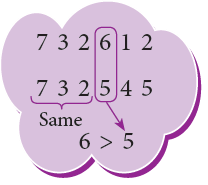Therefore, 7,32,612 > 7,32,545.

How to Write in Ascending and Descending Orders?

• Numbers, when arranged from the smallest to the greatest, are said to be in ascending order.
• Numbers, when arranged from the greatest to the smallest, are said to be in descending order.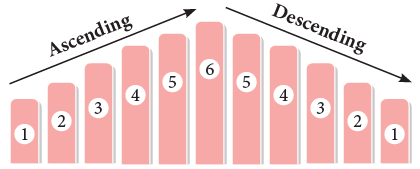Example 1: Arrange the following numbers in ascending order.
12,675 6,532 62,392 18,125 29,358

The smallest number is 6,532. The next number greater than 6,532 is 12,675. The next number greater than 12,675 is 18,125. The other numbers greater than 18,125 in order are 29,358 and 62,392.
∴ 6,532 < 12,675 < 18,125 < 29,358 < 62,392.
Hence, the above numbers when arranged in ascending order are:
6,532  12,675   18,125   29,358  62,392.

What is Successor and Predecessor?

• The successor of a whole number is the number obtained by adding 1 to it. So, the successor of 0 is 1, the successor of 1 is 2, and so on.
• The predecessor of a whole number is one less than the given number. So, the predecessor of 1 is 0, the predecessor of 2 is 1, and so on.
Look at some examples of successor and predecessor of a number: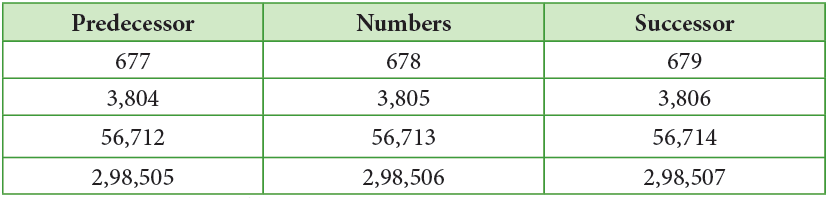How to Form Numbers using the Given Digits?

1. Smallest Number

To write the smallest number using the given digits, arrange the digits in ascending order.

• If 0 is one of the digits, it takes the second place after the smallest digit.
• If two of the given digits are equal, then write them one after the other.

2. Greatest Number

To write the greatest number using the given digits, arrange the digits in descending order.

• If two of the given digits are equal, write them one after the other.

Edurev Tips: Zero occupies the second highest position in forming the smallest number.

Example 1: Use 6, 2, 1, 8 to build the greatest and the smallest 4-digit numbers.

Greatest number: Arranging the digits in descending order, we get 8,621.
Smallest number: Arranging the digits in ascending order, we get 1,268.

Example 2: Use 1, 5, 0, 8, 3 to build the greatest and the smallest 5-digit numbers.

Greatest number: 85,310, Smallest number: 10,358

How to Round off the Numbers?

In our daily life, we hear statements like the following:

• Sarita said that she weighs about 40 kg. She actually weighs 41 kg.
• Mr Dubey paid ₹ 675 for a coat. To his friend he said, “The coat cost me nearly ₹ 700.”
• About 25,000 people watched the IPL match. In fact, 24,750 people watched the match.

In all the statements given above, the given number is being replaced by another number that is “close to” or is an approximation of it. This is called rounding off the given number to a chosen place value. In the first statement, the given number is being rounded off to the nearest ten and in the second and third statements, the given number is being rounded off to the nearest hundred.

1. Rounding off the Numbers to the Nearest Ten

To round off a number to the nearest ten, we round it off to the multiple of ten nearest to it.

In general, for rounding off a number to the nearest ten, look at the digit in the ones place. If it is 1, 2, 3 or 4, then round down the number to the lesser ten. If the digit is 5, 6, 7, 8 or 9, then round up the number to the greater ten.
Example:

• 57 is rounded off to 60. Ones digit is 7, so round up to the greater ten.
• 512 is rounded off to 510. Ones digit is 2, so round down to the lesser ten.
• 1,96is rounded off to 1,970.
• 12,78is rounded off to 12,790.

2. Rounding off the Numbers to the Nearest Hundred

To round off a number to the nearest hundred, we round it off to the multiple of hundred nearest to it.

In general, for rounding off a number to the nearest hundred, look at the digit at the tens place. If it is 1, 2, 3 or 4, round down to the lesser hundred. If the digit is 5, 6, 7, 8 or 9, round up to the greater hundred.
Examples:

• 729 is rounded off to 700. Tens digit is 2, so round down.
• 1,550 is rounded off to 1,600. Tens digit is 5, so round up.
• 24,874 is rounded off to 24,900. Tens digit is 7, so round up.
• 84,214 is rounded off to 84,200.

3. Rounding off the Numbers to the Nearest Thousand

To round off a number to the nearest thousand, we round it off to the multiple of thousand nearest to it.

In general, for rounding off a number to the nearest thousand, look at the digit at the hundreds place. If it is 1, 2, 3 or 4, round down to the lesser thousand. If the digit is 5, 6, 7, 8 or 9, round up to the greater thousand.
Example:

• 8,958 is rounded off to 9,000. Hundreds digit is 9, so round up.
• 16,349 is rounded off to 16,000. Hundreds digit is 3, so round down.
• 29,500 is rounded off to 30,000.
The document Chapter Notes: Introduction to Numbers Notes | Study Mathematics for Class 4 - Class 4 is a part of the Class 4 Course Mathematics for Class 4.
All you need of Class 4 at this link: Class 4Use Code STAYHOME200 and get INR 200 additional OFF

## Mathematics for Class 4

15 videos|68 docs|32 tests

Track your progress, build streaks, highlight & save important lessons and more!

,

,

,

,

,

,

,

,

,

,

,

,

,

,

,

,

,

,

,

,

,

;